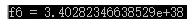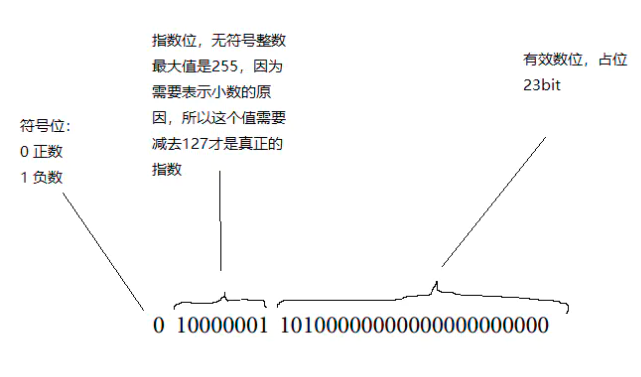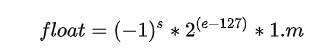m的计算方式是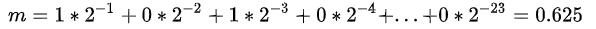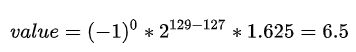`0 01111111 00000000000000000000000`

`0 10000000 0000000000000000000000`

1和2之间，我能表示的数是有限的，比1大的浮点数，最小的值我只能取到

`0 01111111 00000000000000000000001`

`std::numeric_limits<float>::epsilon()`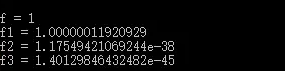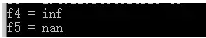``````uint8_t array6 = {0};
array6 = 0x7F; array6 = 0x7F; array6 = 0xFF; array6 = 0xFF;
float* f6 = reinterpret_cast<float*>(array6);
std::cout << "f6 = " << *f6 << std::endl;``````